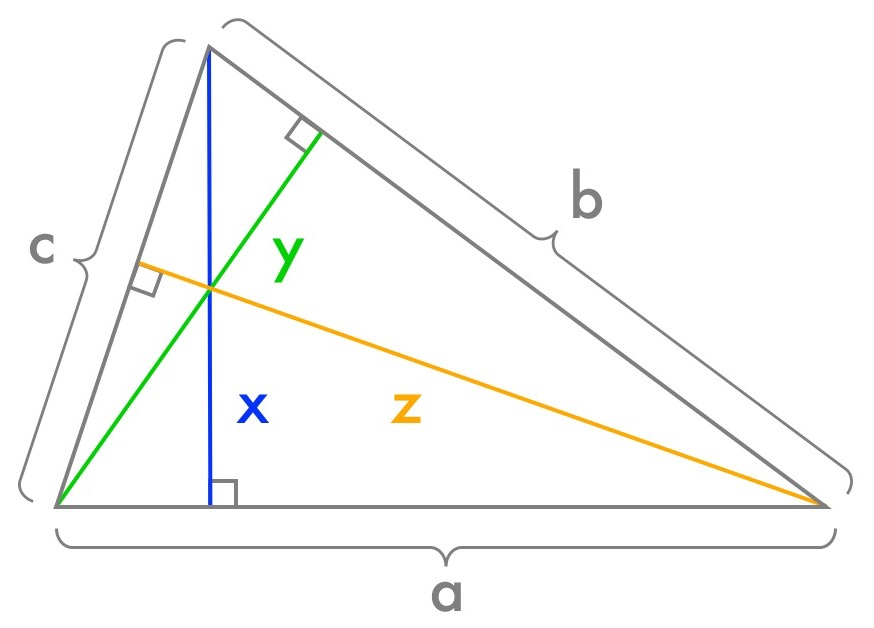# A Triangle of Prime Opportunity - Solution

Let’s call the side lengths $a$, $b$, and $c$, and the altitudes $x$, $y$, and $z$.(By the way, a fun fact: the altitudes of any triangle intersect in a single point called the orthocenter.)

What next? Well, we have altitudes; maybe that’s a clue. So what’s special about altitudes?

One thing that altitudes let us do is very easily calculate the area of the triangle. And since we have all three side lengths and all three altitudes, we can calculate the same area in three different ways:

$$\frac{1}{2}ax = \frac{1}{2}by = \frac{1}{2}cz$$

This is really nice! We want to say something about prime numbers, so having our variables multiplied together is very convenient. And we can cancel all those factors of $\frac{1}{2}$, and give the resulting common value a new variable name $n$:

$$ax = by = cz = n$$

We have three terms – $ax$, $by$, and $cz$ – and we’re trying to fit four prime numbers into them. This sounds like a job for the Pigeonhole Principle! Specifically: if some four of $a$, $b$, $c$, $x$, $y$, and $z$ are prime, then two of those primes must end up in the same term. Let’s say those two primes are $a$ and $x$ (the explanation will follow the same steps if it turns out to be one of the other pairs instead).

Wow. Now we know that $n$ is a number with only two prime divisors. Since $n = ax$, and $a$ and $x$ are both prime, that means the only divisors of $n$ are $1$, $a$, $x$, and $n$. (That’s like the number 6 – its only divisors are 1, 2, 3, and 6.)

Uh-oh. We have six variables ($a$, $b$, $c$, $x$, $y$, and $z$), and only four integer divisors of our number $n$. Time for the Pigeonhole Principle again: some two of those variables have to be the same divisor. But we started out by assuming that $a$, $b$, $c$, $x$, $y$, and $z$ are all different! We have to conclude that we can’t have four of those variables all be prime.

So it turns out Nicki is right; she can use the argument above to explain her reasoning to Dave.